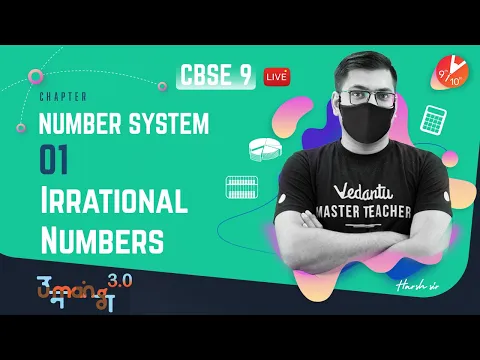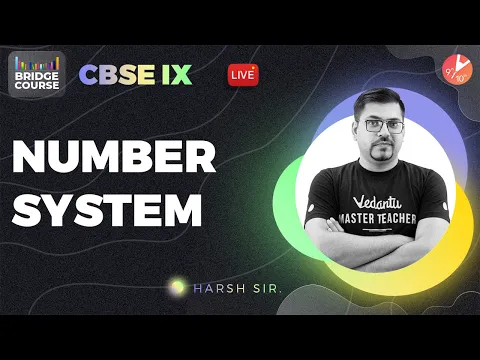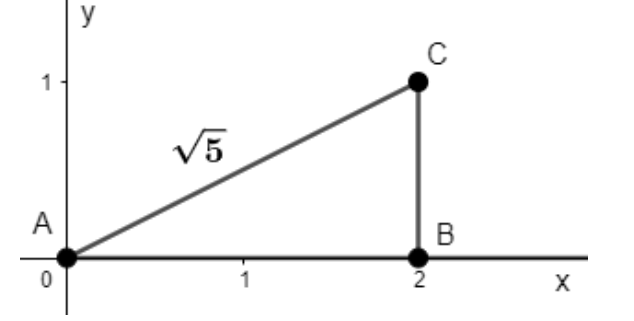Courses
Courses for Kids
Free study material
Offline Centres
More

# NCERT Solutions for Class 9 Maths Chapter 1 - ExerciseLast updated date: 06th Dec 2023
Total views: 534.6k
Views today: 14.34k

## About Exercise 1.2, Chapter 1 - Number Systems

NCERT Solutions for Class 9 Maths Chapter 1 Exercise 1.2 is an elaborative study material for students looking out for in-depth knowledge. The NCERT Maths Class 9 Chapter 1 is written as per NCERT and CBSE guidelines. Many tutors who are very well known names in the field of mathematics have contributed to NCERT solution for Class 9 Maths Chapter 1 to make the chapter easy for students. Vedantu provides students with a Free PDF download option for all the NCERT Solution of updated CBSE textbooks. Subjects like Science, Maths, Engish will become easy to study if you have access to Class 9 Science NCERT Solutions, Maths solutions, and solutions of other subjects that are available on Vedantu only.

NCERT solutions for class 9 maths chapter 1 exercise 1.2 number systems is an exercise designed to teach children more about irrational numbers. If a number cannot be represented as a fraction, it is referred to as an irrational number. As a result, the questions are designed to provide children with a more in-depth understanding of the same concept. NCERT solutions chapter 1 exercise 1.2 consists of four simple problems that help children develop a strong conceptual understanding of irrational numbers.

The number line representation of irrational numbers is an important concept covered in this exercise. Because such numbers cannot be directly marked on a number line, children must develop a thorough understanding of the steps involved.

Watch videos on
NCERT Solutions for Class 9 Maths Chapter 1 - ExerciseNumber System in One Shot | CBSE Class 9 Maths Chapter 1 | CBSE lX - One Shot | Vedantu 9 and 10
Vedantu 9&10
SubscribeShare
11.7K likes
263.2K Views
2 years ago
Play QuizNumber System L-1 | Irrational Numbers | CBSE Class 9 Maths Chapter 1 | Umang 2021 | Vedantu 9 & 10
Vedantu 9&10
9K likes
187.7K Views
2 years agoNumber System | CBSE Class 9 Maths Chapter 1 - Bridge Course 🎯 | Harsh Sir | Vedantu 9 and 10
Vedantu 9&10
7.2K likes
144.2K Views
2 years ago

## NCERT Maths Class 9 Chapter 1 – Exercise 1.2

### Tips to Study with Full Concentration

Here are a few tips for you that will help you in concentrating more on your studies.

• Before you start studying, meditate for 15 - 30 minutes. This will help you in concentrating better.

• Once you start studying, move from topic to topic. Finish one topic at first and then move to the other. Learning is a slow process.

• Practice as much as you can. Practice will make you perfect.

• Drink enough water in between your studies.

• Keep taking small meals in between such as nuts, juices, fruits etc.

• Take enough breaks in between your studies.

### Exercise (1.2)

1. State whether the following statements are true or false. Justify your answers.

(i) Every irrational number is a real number.

Ans: Write the irrational numbers and the real numbers in a separate manner.

• The irrational numbers are the numbers that cannot be represented in the form $\dfrac{p}{q},$ where $p$ and $q$ are integers and $q\ne 0.$

For example, $\sqrt{2},3\pi ,\text{ }.011011011...$ are all irrational numbers.

• The real number is the collection of both rational numbers and irrational numbers.

For example, $0,\,\pm \dfrac{1}{2},\,\pm \sqrt{2}\,,\pm \pi ,...$ are all real numbers.

Thus, it is concluded that every irrational number is a real number.

Hence, the given statement is true.

(ii) Every point on the number line is of the form $\sqrt{m}$, where m is a natural number.

Ans: Consider points on a number line to represent negative as well as positive numbers.

Observe that, positive numbers on the number line can be expressed as $\sqrt{1,}\sqrt{1.1,}\sqrt{1.2},\sqrt{1.3},\,...$, but any negative number on the number line cannot be expressed as $\sqrt{-1},\sqrt{-1.1},\sqrt{-1.2},\sqrt{-1.3},...$, because these are not real numbers.

Therefore, it is concluded from here that every number point on the number line is not of the form $\sqrt{m}$, where $m$ is a natural number.

Hence, the given statement is false.

(iii) Every real number is an irrational number.

Ans: Write the irrational numbers and the real numbers in a separate manner.

• The irrational numbers are the numbers that cannot be represented in the form $\dfrac{p}{q},$ where $p$ and $q$ are integers and $q\ne 0.$

For example, $\sqrt{2},3\pi ,\text{ }.011011011...$ are all irrational numbers.

• Real numbers are the collection of rational numbers (Ex: $\dfrac{1}{2},\dfrac{2}{3},\dfrac{3}{5},\dfrac{5}{7},$……) and the irrational numbers (Ex: $\sqrt{2},3\pi ,\text{ }.011011011...$).

Therefore, it can be concluded that every irrational number is a real number, but every real number cannot be an irrational number.

Hence, the given statement is false.

2. Are the square roots of all positive integer numbers irrational? If not, provide an example of the square root of a number that is not an irrational number.

Ans: Square root of every positive integer does not give an integer.

For example: $\sqrt{2},\sqrt{3,}\sqrt{5},\sqrt{6},...$ are not integers, and hence these are irrational numbers. But $\sqrt{4}$ gives $\pm 2$ , these are integers and so, $\sqrt{4}$ is not an irrational number.

Therefore, it is concluded that the square root of every positive integer is not an irrational number.

3. Represent $\sqrt{5}$ on the number line.

Ans: Follow the procedures to get $\sqrt{5}$ on the number line.

• Firstly, Draw a line segment $AB$ of $2$ unit on the number line.

• Secondly, draw a perpendicular line segment $BC$ at $B$ of $1$ units.

• Thirdly, join the points $C$ and $A$, to form a line segment $AC$.

• Fourthly, apply the Pythagoras Theorem as

\begin{align} & A{{C}^{2}}=A{{B}^{2}}+B{{C}^{2}} \\ & A{{C}^{2}}={{2}^{2}}+{{1}^{2}} \\ & A{{C}^{2}}=4+1=5 \\ & AC=\sqrt{5} \\ \end{align}

• Finally, draw the arc $ACD$, to find the number $\sqrt{5}$ on the number line as given in the diagram below.### NCERT Maths Class 9 Chapter 1 – Exercise 1.2

Chapter 1 Maths Class 9 is the number system. Number system forms the foundation of subsequent understanding of the subject Maths for students. This chapter deals with various kinds of number system according to your syllabus. The number system is the base on whose understanding stands the entire world of Maths. There it is necessary to understand them in detail to score well in the exam. These solutions by Vedantu are available as a free PDF download.

There are various different types of number system. The different types are as follows:

• Natural Numbers - these numbers are used for counting and always starts with one.

• Whole Numbers - this is the set of all-natural numbers including zero

• Integers - the set of all whole numbers and their respective negative numbers is called the integers.

• Rational Numbers - any number that can be written in the form of a ratio of two natural numbers is a rational number.

• Irrational Numbers - any number that cannot be written in the form of a ratio of two natural numbers is irrational.

### Maths NCERT Solutions for Class 9 Chapter 1 Exercise 1.2

This particular exercise in Chapter 1 has a total of four questions to be answered. These questions tend to check the basic understanding of the chapter for the students. This question tests your understanding of all the theories and properties of the chapter of natural numbers.

The number system Class 9 covers all the basic concepts of number system which is eventually useful for other chapters like sets. The whole concept of real numbers. Whole numbers and the entire number system will be a part of your study journey forever. They are the foundation on which the entire branch of arithmetic math will be eventually built. Get the Class 9th Maths Chapter 1 NCERT solutions to ensure you understand all these concepts in detail.

## FAQs on NCERT Solutions for Class 9 Maths Chapter 1 - Exercise

1. Why should I opt for Vedantu’s NCERT solutions for class 9 maths Chapter 1 Exercise 1.2?

NCERT solutions for class 9 maths chapter 1 – Number Systems Exercise 1.2 is the second exercise of Chapter 1 of class 9 Maths. This exercise deals with Irrational numbers especially. Below are the advantages of opting for Vedantu’s NCERT Solutions.

• These NCERT Solutions help you solve and revise all the questions from exercise 1.2.in a very less time.

• After going through the stepwise solutions given by our subject expert teachers, you will be able to get more marks.

• First and foremost, these solutions will help the students score the highest possible marks.

• These are designed as per the NCERT guidelines which help in preparing the Class 9 students accordingly.

• The solutions consist of answers to all the important questions from the final examination point of view.

2. What is Class 9 maths Chapter 1 Exercise 1.2 all about?

NCERT Solutions Class 9 Maths Chapter 1 Number Systems Exercise 1.2 offered by Vedantu are prepared by our subject matter experts which makes it easy for the CBSE board students to learn efficiently. The students refer to these while solving the exercise problems. Exercise 1.2 or the second exercise in Number Systems deals with the irrational numbers. These provide an in-depth and stepwise explanation of each of the questions given in the exercises in the NCERT textbook for class 9 chapter 2. The solutions are prepared as per the latest NCERT curriculum and guidelines so that it should cover the whole syllabus accordingly. These are very helpful in scoring the best possible marks in the examinations.

3. How many questions are there in Class 9 maths Chapter 1 Exercise 1.2?

Class 9 Maths Chapter 1 Exercise 1.2 of the NCERT textbook consists of four questions in total. Among which, three are long type questions and one short question. Our Vedantu solutions consist of answers to all these questions crafted by our excellent experts.

You can download the NCERT solutions Class 9 maths Chapter 1 Exercise 1.2 from Vedantu website and mobile application. These solutions which are provided in PDF format are prepared by our subject matter experts. The students can always use these for reference while solving the exercise problems.

5. What are the topics covered in Exercise 1.2 of Chapter 1 of Class 9 Maths?

There are seven topics covered in Chapter 1 Number System of Class 9 Maths. But the topic on which Exercise 1.2 is based is:

• Exercise 1.1- Introduction to Number System

• Exercise 1.2 deals with the Introduction to irrational numbers

• Exercise 1.3-Real numbers and their decimal expansion

• Exercise 1.4- Representing real numbers on the number line

• Exercise 1.5- Operations on the real number

• Exercise 1.6- Laws of exponents of real numbers

• Summary of the chapter

Students can refer to the NCERT book of Class 9 Maths to understand these topics thoroughly.

6. How can I understand the Class 9 Maths Chapter 1 Number System Exercise 1.2?

If you want to understand Class 9 Maths Chapter 1 Number System easily, then check out Vedantu. Here, you will find a detailed explanation of the chapter in an understandable format. Moreover, you'll also get answers to the NCERT Maths questions for Class 9 Maths Chapter 1 Exercise 1.2 and numerous mock test papers to help you to understand the important concepts. These are available for free both on Vedantu’s website and on Vedantu’s mobile app.

7. How can I make a study plan for preparing Chapter 1 of Class 9 Maths?

To make a successful study plan for preparing the Chapter 1 Number System given in the Class 9 Maths book, you should follow the given steps:

• Build a schedule balanced with all your daily activities.

• Set a timetable and have a designated study area.

• Limit your study time to two hours on this chapter to ensure you don’t lose your focus. You can increase or decrease the time limit according to your preference. During this time, read all the important concepts, solve questions, etc.

8. State whether the statement is true or false. Every point on the number line is in the form of √m where m is a natural number.

The given statement is false. It is wrong according to the norm ‘ a negative can’t be expressed as square roots.

For example- √16= 4 where 4 is a natural number. On the other hand, √3= 1.73 which is not a natural number. Similarly, negative numbers can also be represented on the number line but we know that a negative number changes into a complex number if we take the root of a negative number. For instance- √-8= 8i where i = √-1. Hence, the statement- every point on the number line is in the form of √m where m is a natural number is wrong.

9. How can I score full marks in Exercise 1.2 of Chapter 1 of Class 9 Maths?

Here are the useful tips to score good marks in Exercise 1.2 of Chapter 1 Number System in Class 9 Maths:

• Keep a notebook for theories, formulas and methods to solve important questions for last moment revision.

• Solve tough mathematical problems by yourself.

• Understand Chapter 1 along with its weightage to filter important exercises.

• Pick out the concepts of the chapter that require extra revision. You can do this by solving different question papers.